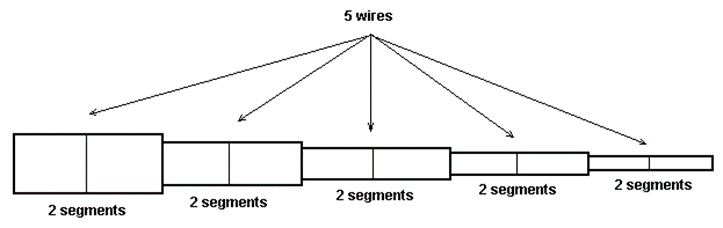## Line

The Line refers to a linear or straight wire. Go to Draw > Line in the main menu to display the Draw dialog box for the Line...

## Arc

The Arc refers to a circular arc. Go to Draw > Arc in the main menu to display the Draw dialog box for the Arc...

## Circle

The Circle refers to a circular loop. Go to Draw > Circle in the main menu to display the Draw dialog box for the Circle...

## Helix

The Helix refers to a helical wire. Go to Draw > Helix in the main menu to display the Draw dialog box for the Helix...

## Archimedean Spiral

The Archimedean Spiral refers to the Archimedes’ spiral with polar equation r(a) = r0 + p/(2p) a, where r0 is the starting radius and p is the pitch. For a spiral with an integer number of turns...

## Logarithmic Spiral

The Logarithmic Spiral refers to a spiral with polar equation r(a) = r0 exp(ba), where r0 is the starting radius (r at a = 0), b = p/(2pr0) and p is the starting pitch...## Tapered Wires

A tapered wire is a wire with a variable radius along its length. The cross section of tapered wires is always circular. The radius is varied linearly along the wire and in defined steps, then a wire with a stepped radius is obtained...